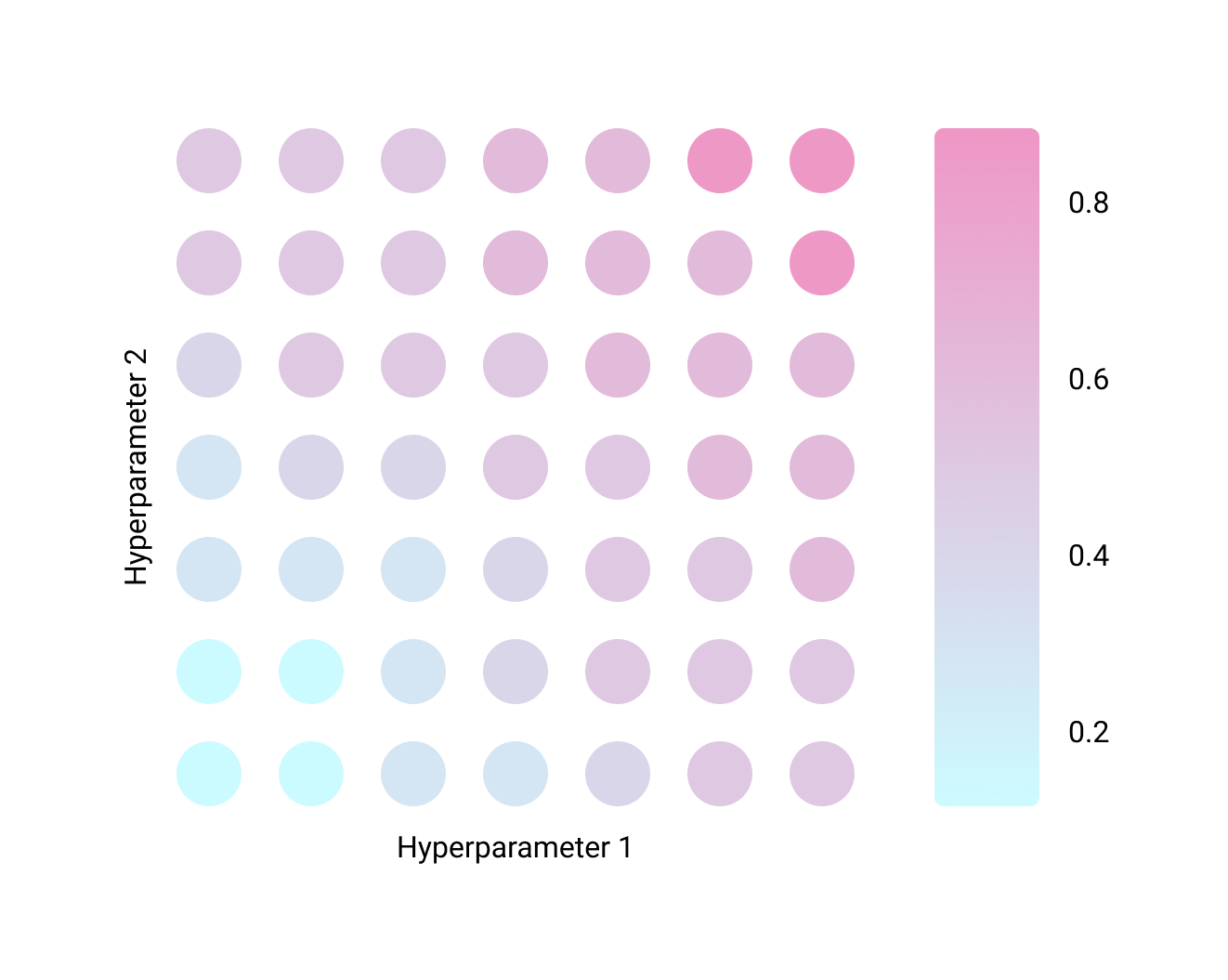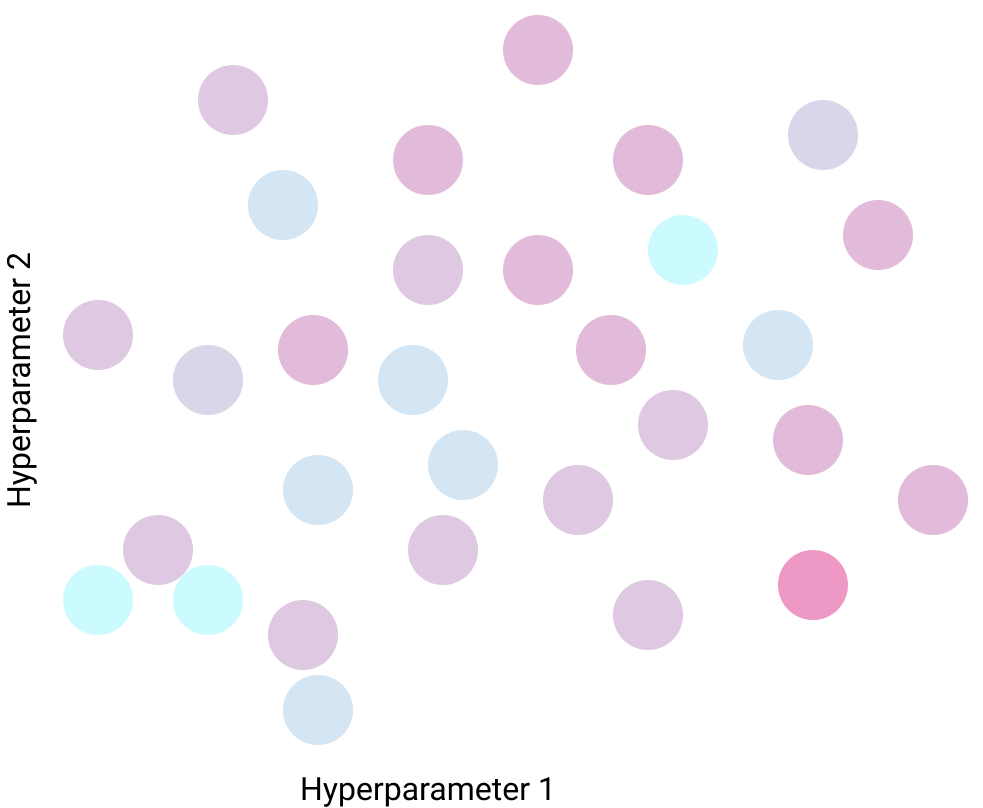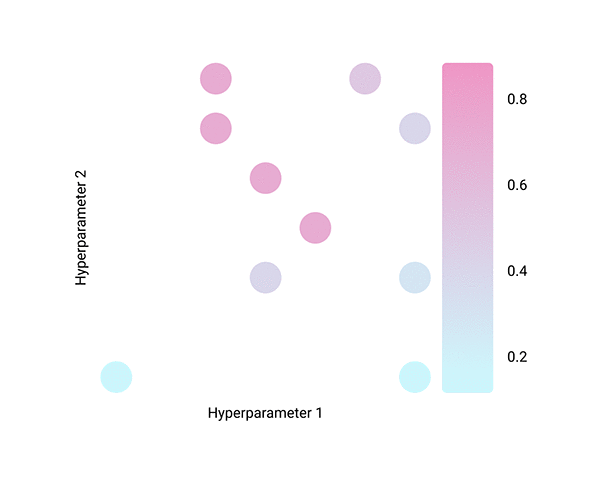Machine Learning Knowledge Center

# Hyperparameter Optimization

## Overview

In this section, we introduce typical hyperparameter optimization methods in machine learning.
The methods described here can be tried from VARISTA.
If you want to know more about hyperparameters, please refer to the following.
What are hyperparameters?

This is a method of selecting the best value by evaluating the score in each grid, using a grid as the search area.
For all combinations, the correct answer rate is calculated and the parameter combination with the highest correct answer rate is adopted.
The correct answer rate is obtained by cross-validation.

However, since all patterns in the search range are tested, the computational cost becomes very high.
For example, if there are two parameters and 20 candidates each, 400 trials are required.
The following is an image of a grid search.Try all patterns within the search range, so you don't miss the best solution.
It is necessary to have an idea of the search range.
Depending on the search range, it may take a long time to search, so it is not suitable for large scale data.

This is a method to evaluate scores by randomly sampling from the parameter space.
Since the combination of parameters to be evaluated is determined based on random numbers, all evaluations can be done asynchronously in parallel.
A comparison between grid search and random search can be shown in the figure below.Doesn't take long to search even when there are many values to adjust.
It is possible to miss something because of random trials.

### Bayes Search - Bayesian Optimization

Bayesian optimization is a successful method in hyperparameter optimization and has been actively studied in recent years. As a result, there are many different methods of Bayesian optimization.

Bayesian optimization is a method that searches for parameters sequentially and stochastically.
In order to find the most optimal combination of parameters, the search focuses on the area where the rate of correct answers is likely to be high, and the search is moderate in areas where there is little search so that the search does not become localized.

The figure below shows the image of Bayesian search.Tends to reduce the number of evaluations, which may reduce computation time.
Difficult to parallelize

#### Some examples of Bayesian optimization methods and open source software

• MTBO: Ax
• TPE: Optuna
• Successive Halving: Optuna
• GP-EI: GpvOpt
• CMA-ES: pycma
• Hyperband: Ray
• PBT: Ray
• BOHB: HpBandSter

## Hyperparameter search performed in VARISTA

VARISTA supports grid search, random search, and Bayesian optimization (Optuna, Hyperopt).

VARISTA is a no-code machine learning tool for engineers that can also be used for free.
It is easy to try because it can be operated by GUI, so please give it a try.

VARISTAは機械学習モデルの開発、管理をノーコードで効率的に行うことができる新しいプラットフォームです。
データをお持ちでしたらすぐに始められますので、是非ともお試しください。

or

Stay in Touch